Mobile QR Code1. (Hanwha systems, Seongnam, 13524, Korea.)
2. (Korea Aerospace University, Goyang, 412-791, Korea.)

Mixer, 25% duty generator, CMOS

## I. INTRODUCTION

A passive mixer is a suitable circuit for receivers, especially for direct conversion receivers (DCR). The flicker noise of a passive mixer is relatively lower than an active mixer because no current flows through a device in switching core (1,2). Moreover, a passive mixer is appropriate for a receiver, which is supposed to tolerate blockers well, because it has better linearity than an active mixer.

Fig. 1(a) shows generally used conventional passive down-conversion mixer with 25% duty LO generator (25DLG). LO$_{0}$, LO$_{90}$, LO$_{180}$, LO$_{270}$ are LO signals with 50% duty whose phases differ by 90 degrees, and LO$_{IP}$, LO$_{IN}$, LO$_{QP}$, LO$_{QN}$ with 25% duty are generated by passing through 25DLG. These signals are then applied to gate of MOSFET in switching core of conventional mixer. When the M1 transistor is turned on by LO$_{IP}$, M5 and M6 are perfectly turned off because LO$_{IP}$ and LO$_{QP}$ have no overlap time. If LO$_{IP}$ and LO$_{QP}$ have 50% duty, M5 is also turned on during the overlap time of LO$_{IP}$ and LO$_{QP}$. Therefore, the M5 transistor can generate unwanted thermal noise due to the formation of on-resistance through node to ground. It also generates flicker noise by creating an unwanted current path. Additionally, a DC offset occurs at the outputs of TIA. For these reasons, LO signal with 25% duty provides better performance than 50% duty.

Fig. 1(b) shows how LO$_{IP}$ is generated. 25DLG is composed of AND gates which decrease the duty of LO signals from 50% to 25%. Consequently, the overlap time is eliminated and the contribution of thermal noise, offset voltage, and flicker noise becomes negligible (3,4). However, 25DLG increases the area of usage and current consumption. Generally, LO signal with 25% duty can be generated by passing the signals of 2LO and LO frequency through the AND gates or by passing the logically operated high-speed divide-by-2 with AND gates for edge-combining (5,6). Thus, these AND gates increase power consumption and size of chip area.

Fig. 1. (a) Block diagram of conventional passive down-conversion mixer with 25% duty LO generator (25DLG), (b) operating principle of 25DLG to generate 25% duty LO.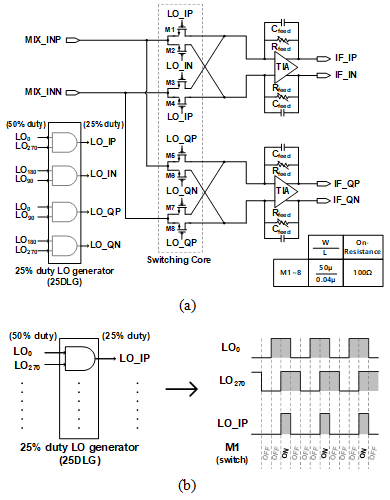Fig. 2(a) shows a quadrature sampling mixer. A quadrature sampling mixer has merit to eliminate 25DLG composed of AND gates. This topology generates 25% duty LO signals by ANDing two 50% duty LO signals with a relative delay of 1/4 period (7,8). Thus, it can reduce current consumption and chip area by 25DLG. The size of MOSFETs in Fig. 2(a) to achieve the same performance of conventional mixer should be double for making on-resistance of MOSFETs same. In addition, each LO signal should drive at least three MOSFETs in Fig. 2(a). Therefore, each LO buffer need additional power consumption.

Fig. 2(b) shows proposed mixer switching core. It operates in a similar way to quadrature sampling mixer and has the same size MOSFETs as quadrature sampling mixer. However, the proposed mixer has merit in power consumption and occupying area because it need only 2/3 of the MOSFETs compared to the quadrature sampling mixer.

Fig. 2. (a) Quadrature sampling mixer(single-ended input used) (7,8), (b) Proposed mixer switching core(single-ended input used).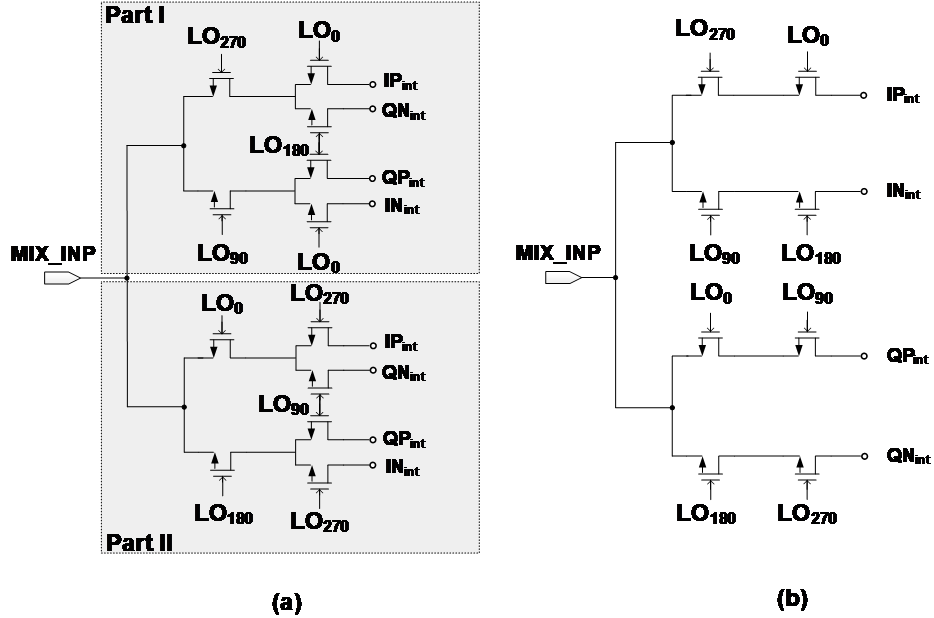In Fig. 3(a), new duty-generator-less mixer is proposed. The proposed mixer does not require AND gates which perform the edge-combining of LO signals with 50% duty. By adding only one additional switch to each MOSFET of conventional switching core in series, the performance of a proposed mixer becomes equivalent to that of a mixer with 25DLG. The series switch, which replaces AND gate, reduces power consumption and saves occupying area for 25DLG. These are advantages.

Fig. 3. (a) Block diagram of proposed duty-generator-less passive down-conversion mixer, (b) operating principle of generating of 25% duty LO.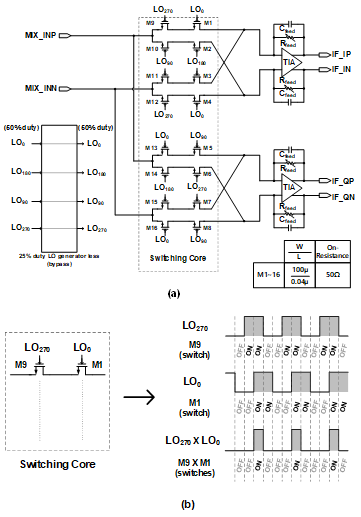Fig. 4. (a) Block diagram of proposed mixer, (b) timing diagram of LO wave form.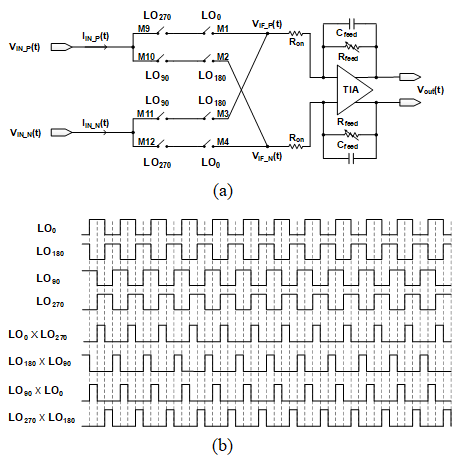## II. PROPOSED DUTY-GENERATOR-LESS PASSIVE MIXER

Switching core of proposed mixer is composed of additional switch directly connecting to each MOSFET in switching core of conventional mixer. It replaces the 25DLG. In Fig. 3(a), MIX_INP and MIX_INN, the input voltage signals of the mixer, are converted to the current signals when the series connected switches are turned on simultaneously. In Fig. 3(b), during the overlapped time of LO$_{270}$ and LO$_{0}$, M9 and M1 switches are turned on simultaneously and then the input signal passes through M9 and M1 switches. Therefore, this operation is equivalent to the operation of M1 switch by LO$_{IP}$ with 25% duty in Fig. 1(b). However, the structure of switch is more complex. Even though LO buffers of proposed mixer use more current than that of conventional mixer, the proposed mixer uses less current and saves space because it doesn’t have 25DLG.

The LO$_{0}$, LO$_{90}$, LO$_{180}$, and LO$_{270}$ are LO signals with 50% duty. The overlapped time of LO$_{0}$ and LO$_{270}$ produces LO signal with 25% duty at 0 degrees. The overlapped time of LO$_{180}$ and LO$_{90}$ creates LO signal with 25% duty at 180 degrees. The quadrature signals can be generated by a different combination in Fig. 4(b). The next stage of switching core is TIA with RC feedback. The current signal after switching core is converted to voltage signal after TIA.

A signal is down-converted and decreased while passing through switches and it is amplified by the TIA with RC feedback connected to the backside of switches. In Fig. 5, the two-stage inverter is employed for the structure of TIA. The open loop gain is 49 dB and phase margin is 65 degree. The P1dB (1dB Gain Compression Point) of the entire circuit of this study is determined by the output of the TIA. TIA has the VDD of 1.8 V and uses two stacks. Therefore, when the overdrive voltage is assumed to be 250 mV per stack, the maximum voltage swing range is approximately 1.3 V$_{\mathrm{o-p}}$(zero to peak). Consequently, OP1dB (Output 1dB Gain Compression Point) of proposed mixer becomes approximately 12 dBm.

### 1. Gain Analysis of Proposed Mixer

At Fig. 4(b), each waveform of LO signals needs to be evaluated with Fourier transform for calculating the conversion gain (9). In these waveforms, LO$_{0}$ is expressed as a switching function and written as;

##### (1)
\begin{align} \begin{array}{l} g_{L{O_{0}}}=a_{0}+\sum _{n=1}^{\infty }\frac{2}{n\pi }\sin \left(n\pi \eta _{0}\right)\cos \left(\frac{2n\pi t}{T}\right)\\ =\eta _{0}+\frac{2}{\pi }\cos \left(\omega _{LO}t\right)- \frac{2}{3\pi }\cos \left(3\omega _{LO}t\right)+\frac{2}{5\pi }\cos \left(5\omega _{LO}t\right)- \ldots \end{array} \end{align}

where $\eta_{0}$ is the duty of LO$_{0}$. In the same method, the other switching function is expressed as;

##### (2)
$$g_{L O_{180}}=\eta_{180}-\frac{2}{\pi} \cos \left(\omega_{L O} t\right)+\frac{2}{3 \pi} \cos \left(3 \omega_{L O} t\right)-\frac{2}{5 \pi} \cos \left(5 \omega_{L O} t\right)+\mathrm{L} \ldots$$

##### (3)
$$g_{L O_{90}}=\eta_{90}-\frac{2}{\pi} \sin \left(\omega_{L O} t\right)+\frac{2}{3 \pi} \sin \left(3 \omega_{L O} t\right)-\frac{2}{5 \pi} \sin \left(5 \omega_{L O} t\right)+L_{\cdots}$$

##### (4)
$$g_{L O_{270}}=\eta_{270}+\frac{2}{\pi} \sin \left(\omega_{L O} t\right)-\frac{2}{3 \pi} \sin \left(3 \omega_{L O} t\right)+\frac{2}{5 \pi} \sin \left(5 \omega_{L O} t\right)-\mathrm{L} \ldots$$

where $\eta_{180}$, $\eta_{90}$, and $\eta_{270}$ are the duties of LO$_{180}$, LO$_{90}$, and LO$_{270}$, respectively. When these duties of LO signals are assumed to be 50%, the ideal condition, $\eta_{0}$, $\eta_{90}$, $\eta_{180}$, and $\eta_{270}$ are approximately 0.5.

For easy calculation, the voltage conversion gain (G$_{\mathrm{mix}}$) in Fig. 4(a) can be written as Eq. (5);

##### (5)
$G_{mix}=\frac{V_{IF\_ P}(t)- V_{IF\_ N}(t)}{V_{IN\_ P}(t)- V_{IN\_ N}(t)}$

$V_{I F_{-} P}(t)$ and $V_{I F_{-} N}(t)$ can be written as;

##### (6)
\begin{equation*} V_{IF\_ P}\left(t\right)=V_{IN\_ P}\left(t\right)g_{L{O_{270}}}g_{L{O_{0}}}+V_{IN\_ N}\left(t\right)g_{L{O_{90}}}g_{L{O_{180}}} \end{equation*}

##### (7)
\begin{equation*} V_{IF\_ N}\left(t\right)=V_{IN\_ N}\left(t\right)g_{L{O_{270}}}g_{L{O_{0}}}+V_{IN\_ P}\left(t\right)g_{L{O_{90}}}g_{L{O_{180}}} \end{equation*}

where $V_{I N_{-} P}(t)=-V_{I N_{-} N}(t)=A_{R F} \cos \left(\omega_{R F} t\right)$. Eqs. (6, 7) into Eq. (5), G$_{\mathrm{mix}}$ is simplified as follows.

In the switch function, harmonics above the third order can be negligible. The conversion gain, G$_{\mathrm{mix}}$ converges to$\sqrt{2}/\pi$ by Eq. (8). The final transfer function of the proposed mixer can be obtained as follows. The feedback capacitance, C$_{\mathrm{feed}}$ of RC feedback can be negligible in this gain calculation.

##### (9)
\begin{align} \begin{array}{l} A_{V}=G_{mix}\times \frac{V_{out}\left(t\right)}{V_{IF\_ P}- V_{IF\_ N}\left(t\right)}\\ \cong - \frac{\sqrt{2}}{\pi }\times \frac{R_{feed}}{R_{on}}\left[1- \frac{1}{A_{o}}\left(1+\frac{R_{feed}}{R_{on}}\right)\right] \end{array} \end{align}

where A$_{\mathrm{o}}$ is the open-loop gain of TIA, R$_{\mathrm{feed}}$ is the feedback resistance of TIA with RC feedback, and R$_{\mathrm{on}}$ is the on resistance of series connected switches in the mixer. The value in square brackets in Eq. (9)can be replaced by 1 because A$_{\mathrm{o}}$ is very high.

### 2. Noise Analysis of Proposed Mixer

The four noise sources are observed as output noise at the output node in Fig. 6. The thermal noise in RF frequency, which are contributed by the input resistance (R$_{\mathrm{s}}$), are down-converted to the output noise with IF frequency. And the thermal noise of on resistance (R$_{\mathrm{on}}$) of the switches is presented output node of switching core. The feedback resistance (R$_{\mathrm{feed}}$) of TIA with RC feedback and the input referred noise voltage (V$_{\mathrm{n,inop}}$) of TIA are contributed to the output of the proposed mixer (4, 10, 12). Although the noises of TIA are composed of thermal noise and flicker noise, the flicker noise is negligible in the frequency of more than 100 Hz. Therefore, the flicker noise is ignored in this calculation.

Fig. 7. Noise components converge to IF frequency by LO harmonics.The circuit in Fig. 6 can be converted to half circuit. From analysis of half circuit, the output noise power is evaluated as;

##### (10)
\begin{align} \begin{array}{l} \overline{{V_{n,out}}^{2}\left(t\right)}=4KTR_{s}\left(\frac{2R_{feed}}{R_{s}G_{mix}+2R_{on}}\right)^{2}+8KTR_{on}\left(\frac{R_{feed}}{R_{on}}\right)^{2}\\ +8KTR_{feed}+\overline{V_{n,inop}\left(t\right)^{2}}\left(\frac{R_{s}G_{mix}+2R_{on}+2R_{feed}}{R_{s}G_{mix}+2R_{on}}\right)^{2} \end{array} \end{align}

Noise is presented by power because it appears randomly. A conversion gain (G$_{\mathrm{mix}}$) from RF port to output node of switching core is$\sqrt{2}/\pi$ by Eq. (8)since above third harmonic of the switch function could be ignored. In the case of noise, however, the harmonics cannot be ignored because the thermal noise distributed over the entire frequency band is down-converted to the IF frequency by LO’s harmonic components. The noise contribution of harmonics above third-order is about 10% (11). Therefore, the noise contribution by harmonics should be included in calculation. As a result, conversion gain for noise, G$_{\mathrm{mix}}$ converges to$1/2$ instead of $\sqrt{2}/\pi$. It can be proved by following equations.

##### (11)
\begin{aligned} G_{\operatorname{mix}} &=\frac{V_{I F_{-} P}(t)-V_{I F_{-} N}(t)}{V_{I N_{-} P}(t)-V_{I N_{-} N}(t)} \\ &=\frac{\sqrt{2}}{\pi} \cdot\left(1 \cdot \frac{\cos \left(\omega_{I F} t+\frac{\pi}{4}\right)}{\cos \left(\omega_{R F} t\right)}-\frac{1}{3} \cdot \frac{\cos \left(\omega_{I F} t+\frac{\pi}{4}\right)}{\cos \left(\omega_{3 R F} t\right)}+\frac{1}{5} \cdot \frac{\cos \left(\omega_{I F} t+\frac{\pi}{4}\right)}{\cos \left(\omega_{5 R F} t\right)}+\ldots\right) \end{aligned}

where $V_{N_{-} P} (t)=-V_{I N_{-} N}(t)=A_{N} \cos \left(\omega_{A L L} t\right)$. In the switch function, harmonics can’t be negligible. $A_{N}$ is the amplitude of noise and $\omega_{ALL}$ means the entire frequency band. $\omega_{3RF}$, $\omega_{5RF}$, and more components included in $\omega_{ALL}$ converge to $\omega_{IF}$ by the harmonics of the LO frequency in Fig. 7.

Fig. 8. Open loop gain and input referred noise voltage of TIA.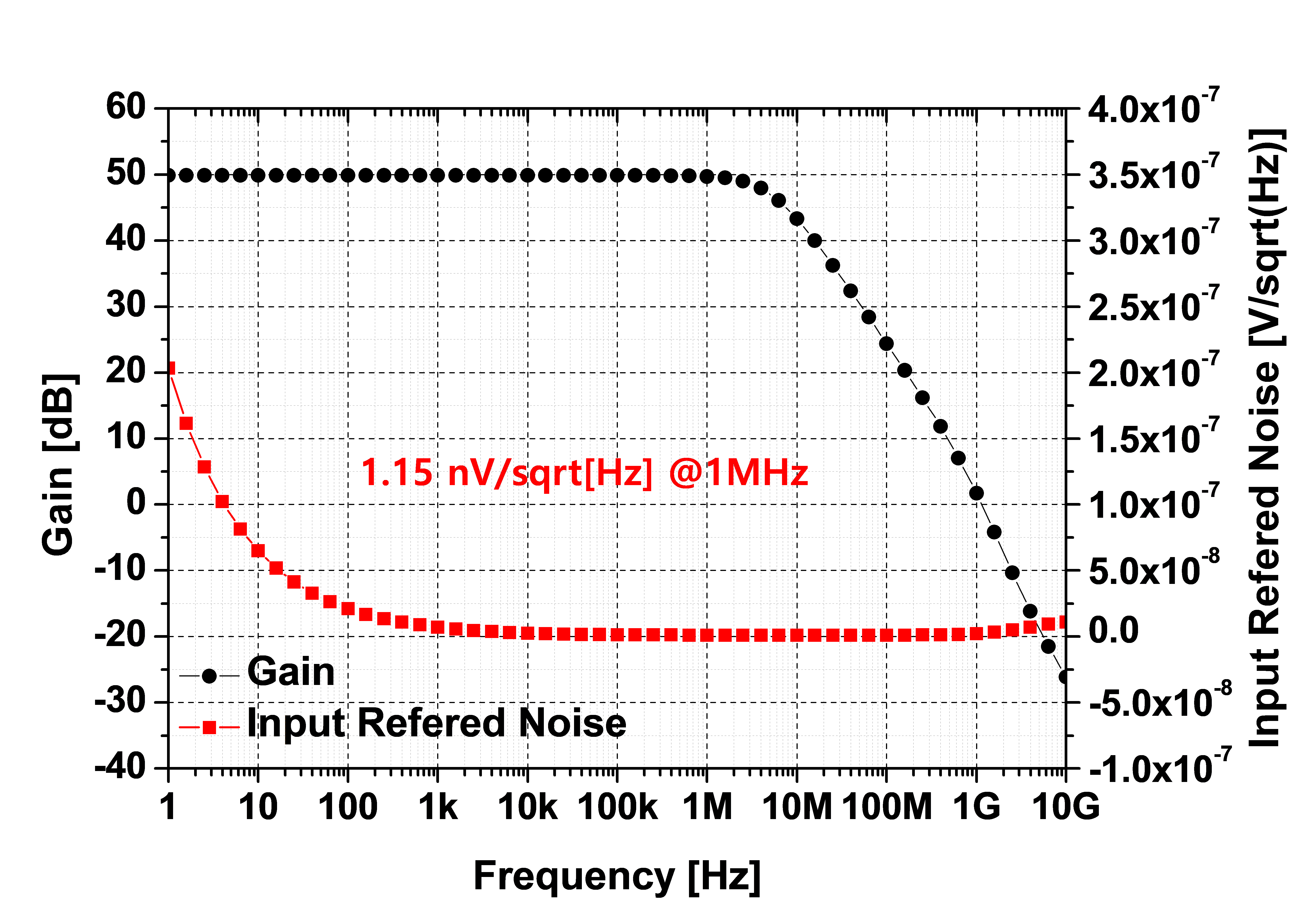Since noise is generally represented as a square and each term of Eq. (11)is independent, (G$_{\mathrm{mix}}$)$^{2}$ can be expressed as;

##### (12)
\begin{align} \begin{array}{l} \left(G_{mix}\right)^{2}=\left(\frac{\sqrt{2}}{\pi }\right)^{2}\cdot \left[\left(\frac{1}{1}\right)^{2}+\left(\frac{1}{3}\right)^{2}+\left(\frac{1}{5}\right)^{2}+....\right]\\ =\left(\frac{\sqrt{2}}{\pi }\right)^{2}\frac{\pi ^{2}}{8} \end{array} \end{align}

Therefore, we can see that G$_{\mathrm{mix}}$ coverges to 1/2.

$A_{v, t o t a l}^{2}$ means total gain from input source to the output node in Fig. 6. It is obtained from the following equation.

##### (13)
\begin{equation} {A^{2}}_{V,\textit{total}}=\left(\frac{2R_{feed}}{R_{s}G_{mix}+2R_{on}}\right)^{2}\left[1- \frac{1}{{A_{o}}^{2}}\left(1+\frac{2R_{feed}}{R_{s}G_{mix}+2R_{on}}\right)^{2}\right] \end{equation}

The value in square brackets can be replaced by approximately 1. The Based on the above equations, $\overline{{V_{n,out}}^{2}(t)}$, ${A^{2}}_{V,\textit{total}}$, the final noise can be developed as follows (12).

##### (14)
\begin{align*} \begin{array}{l} NF=\frac{\overline{{V^{2}}_{n,out}\left(t\right)}}{{A^{2}}_{V,\textit{total}}}\cdot \frac{1}{4kTR_{s}}\\ \cong 1+\frac{\left(G_{mix}R_{s}+2R_{on}\right)^{2}}{2R_{S}R_{on}}+\frac{\left(G_{mix}R_{s}+2R_{on}\right)^{2}}{2R_{S}R_{feed}}\\ +\frac{\overline{{V_{n,inop}}^{2}}}{4KTR_{S}}\left(1+\frac{G_{mix}R_{s}+2R_{on}}{2R_{feed}}\right)^{2} \end{array} \end{align*}

Fig. 9. Simulation, measurement, and calculation results of conversion gain.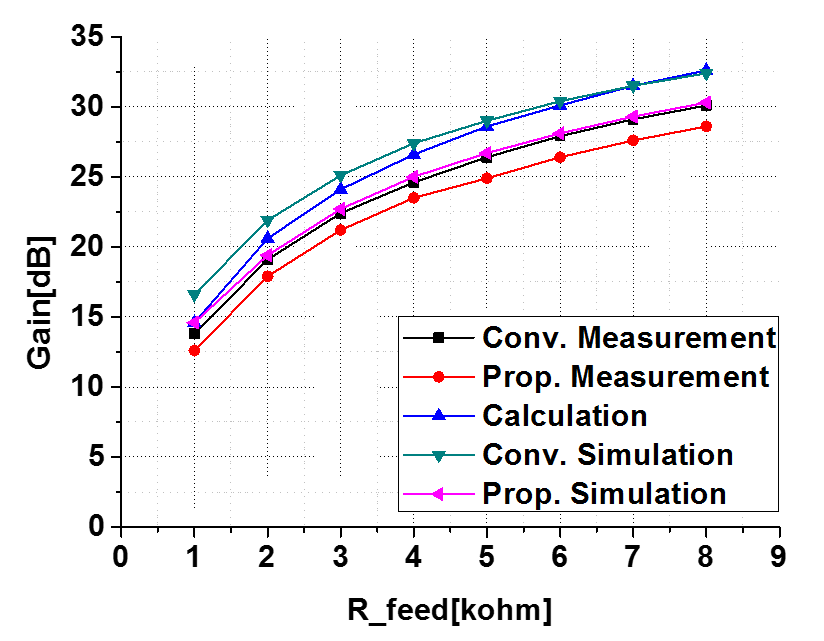where V$_{\mathrm{n,inop}}$ is the equivalent noise voltage at the input of TIA. V$_{\mathrm{n,inop}}$ was simulated to calculate the NF of the proposed mixer in Fig. 8.

## III. MEASUREMENT RESULTS

The simulation and measurement results were compared as a next step. Manual calculation and simulation results were almost identical to the measurements in Fig. 9 and 10. Gain and NF of conventional mixer and proposed mixer were measured between 500 MHz and 2000 MHz in frequency when R$_{\mathrm{feed}}$, feedback resistance of TIA with RC feedback is 4 kohm in Fig. 11. Measured IIP3 (Input third-order Intercept Point) of conventional mixer and proposed mixer were almost identical in Fig. 12. The two-tone test was performed with a difference of 500 kHz from 900~MHz.

The white box located at the center of the die photo represents the mixers including switching core of a conventional mixer, a duty generator, and the switching core of proposed mixer in Fig. 13. The right side of the box is a switching core of a conventional mixer and a duty generator. The left side is a switching core of the proposed mixer. Another white box shows the circuit of TIA with RC feedback. CMOS 40 nm process was used and 1.8 V was used as TIA supply voltage. Moreover, 1.1 V was used as LO buffer supply voltage for the conventional and proposed mixer.

Fig. 11. Measured Gain and NF of conventional mixer and proposed mixer by frequency.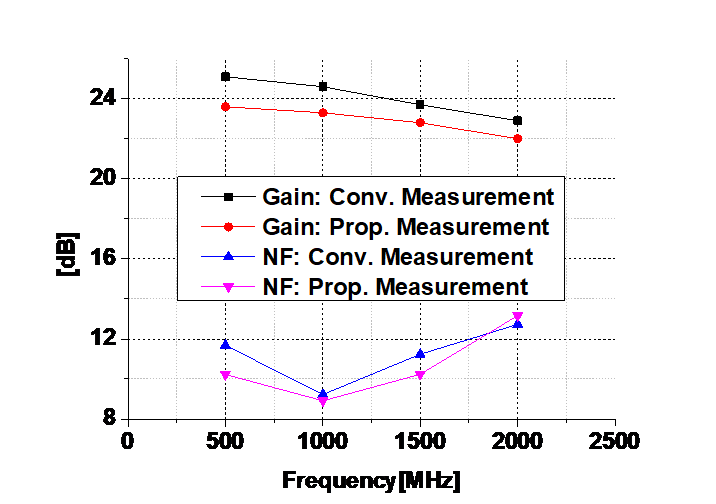Fig. 12. Measured IP3 of conventional mixer and proposed mixer.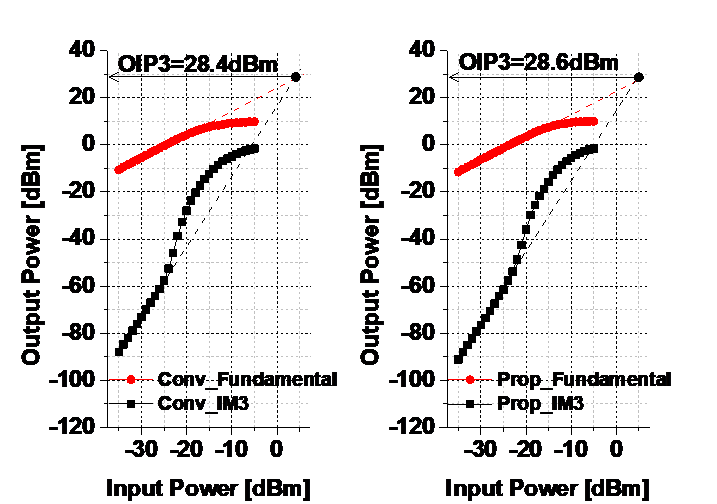Table 1. Performance Comparison

 Calculation Conv. Sim. Conv. Mea. Prop. Sim. Prop. Mea. Unit Process 40 nm CMOS Freq. 900 MHz Gain 27.4 27.4 24.6 24.9 23.6 dB NF 8.3 8.3 9.2 8.6 8.9 dB OP1dB 12.2 12.8 7.9 7.9 7.4 dBm OIP3 22.2** 30.7 28.4 32 28.6 dBm Size* 20214 10274 μm2 Current (Mixer) 6.3 6.3 6.0 6.3 6.0 mA Current (LO) 3.5 ***3.5 ***3.3 ****2.5 ****2.4 mA

* without area by TIA, ** OIP3 = OP1 dB + 10, *** 25 DLG + LO buffers from 1.1 V supply, **** LO buffers from 1.1 V supply

The performance of this circuit was summarized in case of 900MHz frequency in Table 1. However, it is operable from 500 MHz to 2000 MHz. The proposed mixer can save 0.9 mA current consumption and 9940 $\mu \mathrm{m}^{2}$ chip area compared to that of conventional mixer. Moreover, Gain and NF performances were found to be almost identical between two mixers.

The majority of mixers have used a 25DLG to generate LO signals with 25% duty. However, this study successfully generated the LO signal with 25% duty simply by adding only one MOSFET to switch core of conventional mixer in series. The advancement allows us to save the current and area that are required by a 25DLG. Despite these advantages, the performance including Gain and NF does not degrade. Nowadays, the area of a chip is getting smaller to achieve a high-density with using less power. The results of this study will help to advance this trend.

## IV. CONCLUSIONS

The majority of mixers have used a 25DLG to generate LO signals with 25% duty. However, this study successfully generated the LO signal with 25% duty simply by adding only one MOSFET to switch core of conventional mixer in series. The advancement allows us to save the current and area that are required by a 25DLG. Despite these advantages, the performance including Gain and NF does not degrade. Nowadays, the area of a chip is getting smaller to achieve a high-density with using less power. The results of this study will help to advance this trend.

### ACKNOWLEDGMENTS

This work was supported by the Technology Innovation Program (or Industrial Strategic Technology Development Program, 20004325, Low power and Multi Functional CMOS Sensor SoC for Motion Detection and IoT Applications) funded By the Ministry of Trade, Industry&Energy(MOTIE, Korea)

This work was supported by the Electronics and Telecommunications Research Institute (ETRI) grant funded by the Korean government [2019-0-00933, Development on Multi-beam Antenna Technology]

### REFERENCES

1
Zhou S., Chang M. F., May 2005, A CMOS passive mixer with low flicker noise for low-power direct-conversion receiver, IEEE J. Solid-State Circuits, Vol. 40, No. 5, pp. 1084-10932
Hsiao Yu. Chih, Meng Chin Chun, Syu Jin-Siang, Lin Chung-Yo, Wong Shyh-Chyi, Huang Guo-Wei, 2012, 5-6 GHz 9.4 mW CMOS Direct-Conversion Passive-Mixer Receiver With Low- Flicker-Noise Corner, Proceedings of the 7th European Microwave Integrated Circuit Conference3
Lee J., et al , July 2011, A High Linear Low Flicker Noise 25% Duty Cycle LO I/Q Mixer for a FM Radio Receiver, IEEE International Symposium on Circuits and Systems, pp. 1399-14024
Poobuapheun N., Chen W., Zdravko B., Niknejad A. M., Aug 2007, A 1.5-V 0.7–2.5-GHz CMOS quadrature demodulator for multiband direct-conversion receivers, IEEE J. Solid-State Circuits, Vol. 42, No. 8, pp. 1669-16765
Lee D., Lee J., Park H., Choi J., Park S., Kim I., Pu Y., Kim J., Hwang K., Yang Y., Seo M., Lee K., 2016, Design of a Wide-Frequency-Range, Low-Power Transceiver with Automatic Impedance-Matching Calibration for TV-White-Space Application, J. Semiconductor Technology and Science, Vol. 16, pp. 126-1426
Yu X., Fang Y., Shi Z., 2016, 2.5mW 2.73 GHz non-overlapping Multi-phase Clock Generator with Duty-cycle Correction in 0.13m CMOS, Electronics Lett, Vol. 52, pp. 1261-12627
Ikeuchi O., Saito N., Nauta B., June 2010, Quadrature Sampling Mixer Topology for SAW-Less GPS Receivers in 0.18µm CMOS, IEEE Symposium on VLSI, pp. 177-1788
Kim J., Silva-Martines J., Sep 2013, Low-Power, Low-Cost CMOS Direct-Conversion Receiver Front-End for Multistandard Applications, IEEE Journal of Solid-State Circuits, Vol. 48, No. 99
Kim Y., Son Y., Parkhomenko V. N., Hwang I., Cho J., Nah K., Park B., 2005, A GSM/EGSM/DCS/ PCS Direct Conversion Receiver with Integrated Synthesizer, IEEE Trans. Microwave. Theory and Techniques, Vol. 53, pp. 606-61310
Choi C. H., Kwon K. D., Nam I. K., 2016, A 370µW CMOS MedRadio receiver front-end with inverter-based complementary switching mixer, IEEE Microwave and Wireless Components Lett, Vol. 26, pp. 73-7511
Terrovitis M. T., Meyer R. G., 1999, Noise in currentcommutating CMOS mixers,, Vol. 34, pp. 772-78312
Lin Z., Mak P.-I., Martins R. P., 2014, A 0.14-mm2 1.4-mW 59.4-dBSFDR 2.4 GHz ZigBee/WPAN receiver exploiting a split-LNTA + 50% LO topology in 65-nm CMOS, IEEE Trans. on Microwave Theory and Techniques, Vol. 62, pp. 1525-1534## Author

received the B.S., M.S. degrees in the department of electrical engineering, Korea Aero space University, Go-yang, Korea, in 2014, and 2016, respectively.

His M.S. dissertation focused on the Receiver architecture about noise cancelling LNA and 25% duty generator-less mixer.

From 2016, he has been working at Hanwha systems, Seongnam, Korea which engage in defense industry.

He is an engineer with the Electronic optics part, especially engaged in receiver for Laser Spot Tracker (LST) system and has experience of Radar system, making beacon for testing main radar specification.

received the B.S. degree in electrical engineering from the Kyung-pook national university in 1995, the M.S. and Ph.D. degree in electrical engineering from the KAIST (Korea Advanced Instituted of Science and Technology) in 1997 and 2002, respectively.

His Ph.D. dissertation focused on the transceiver architecture about image rejection and spurious rejection.

In 2002, he joined Samsung Electronics, Co., Ltd., Korea, as a Senior Engineer.

Since then he has participated in the design of CDMA and GSM/GPRS wireless mobile application.

Since then he has been designing LNA and down-conversion mixer for multi-mode CDMA and GSM/GPRS.

In 2006, He joined the school of Electronics and Information Engineering, Korea Aerospace University, Seoul, Korea.

received the B.S., degrees in the department of electrical engineering, kwangwoon University, Seoul, Korea, in 2003.

Mr. Han joined the Electro-Optronics 2 Team of Hanwha Systems, Korea, in 2002.

He is currently a Senior Engineer in the Electro-optics R&D Site, Hanwha systems.

He is interested in Analog & Digital Image Processing of Long Wave IR.

received the B.S. degrees in Electronic Engineering from Kyung hee University, Korea, in 2010.

Mr. Shin joined the Electro-Optronics 2Team of Hanwha Systems, Korea, in 2010.

He is currently a Senior Engineer in the Electro-opticsㆍPGM R&D Site, Hanwha systems.

He is interested in Analog & Digital Image Processing of Long Wave IR.

received his B.S. in control and instrumentation engi-neering from Seoul National University in 1987, his M.S. in electrical and computer engineering from the University of South Carolina in 1995, and his Ph.D. in electrical and computer engineering from the University of Colorado in 1998.

From 1987 to 1993, he worked at LG Electronics with a focus on communication systems.

From 1999 to 2003, he worked for Curitel, where he was principally involved with the development of mobile phones.

He joined the School of Electronics and Information Engineering at Korea Aerospace University in 2004.

His research interests include the design of RFIC/MMIC, millimeter-wave ICs, analog circuits, and radar systems.